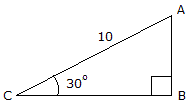# Engineering Mechanics - General Principles - Discussion

### Discussion :: General Principles - General Questions (Q.No.5)

5.Using the basic trigonomic functions, determine the length of side AB of the right triangle.

 [A]. h = 5.77 [B]. h = 11.55 [C]. h = 5 [D]. h = 8.66

Explanation:

No answer description available for this question.

 Sathiya said: (Dec 13, 2010) sin 30 = 0.5 SIN C = OPP / ADJ (c=AB/CA) CA = 10 So 0.5 =AB/10 AB =10*0.5 AB =5

 Suhas said: (Feb 12, 2011) Opposite side to the 30 degree in right angle triangle is half of diagonal.

 Sweety said: (Feb 12, 2011) sin30=AB/10 1/2=AB/10 AB=10/2 AB=5

 Shanavaz said: (Mar 1, 2011) sin 30 = 1/2 sin 30 = AB/10 AB = 5

 Nishita said: (Apr 28, 2011) By using 30, 60, 90 degree formula. We get a ans.

 Rookiemathlete said: (Nov 13, 2011) You can easily use sine law, which is (Side A)/(opposite angle) = (Side B)/(opposite angle) = (Side C)/(opposite angle). in this problem (Side AB)/Sin30 = 10/Sin90 Side AB = (10(Sin30))/Sin90 AB = 5/sin90 AB = 5

 Akanksha said: (Nov 30, 2011) All explanation are correct.

 Koko said: (Feb 20, 2012) sin 30 = 0.5 * ab/bc opp/adj= 0.5 * 10 = 5

 Karmukilan said: (Aug 8, 2012) Basic Sin (theta) =Opp/Hyp. So sin30degree=AB/10. AB=sin30X10. =0.5X10. AB=5 this is the ANSWER.

 Omer Mohammed said: (Dec 30, 2012) AB/sinC = AC/sinB = BC/sinA sinC = sin30= 0.5 sinB = sin90 = 1 So, AB/0.5 = 10/1 0.5 AB = 10 AB = 5.

 Pradeep. said: (Feb 11, 2015) sin30 = ab/ac. 0.5 = ab/10. So ab = 5.

 Vinod Choudhary said: (Sep 10, 2016) Sin 30 = AB/AC, Sin 30 = AB/10 (Because, AC = 10), 1/2 = AB/10 (Because sin 30 = 1/2), 10/2 = AB, AB = 5 = h.